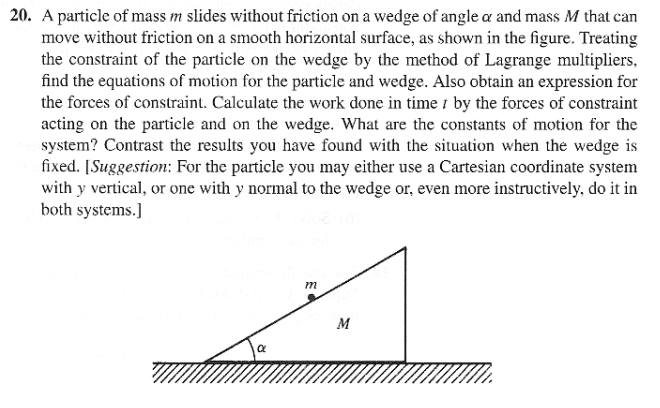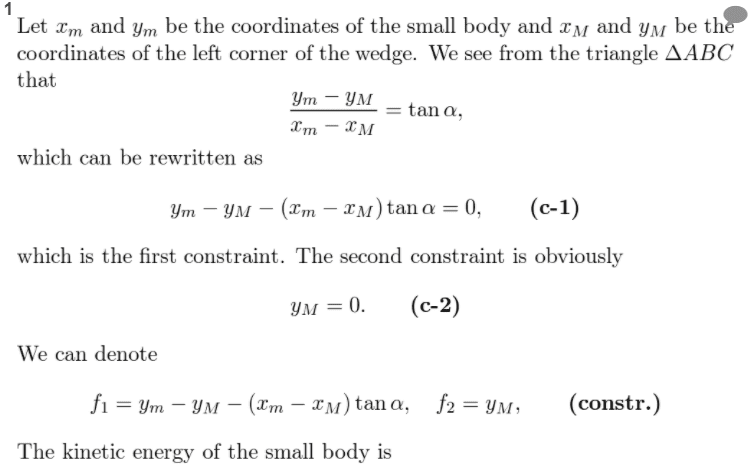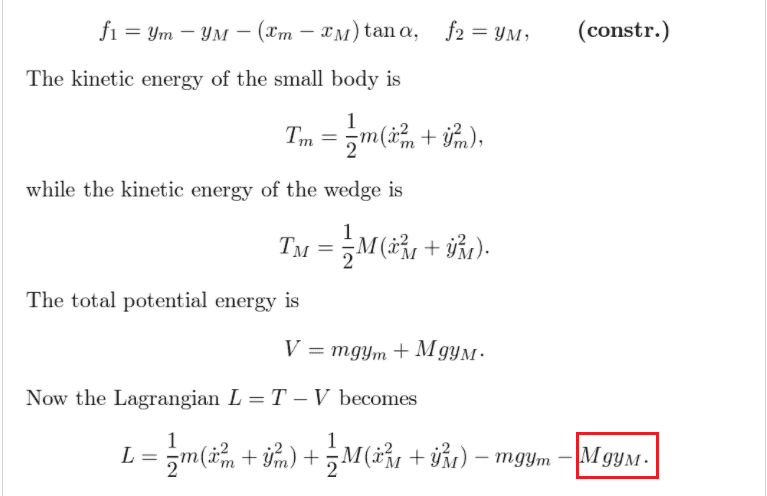Another
Homework Statement:
Find the Lagrangian of system.
In the question say the wedge can move without friction on a smooth surface.
Why does the potential energy of the wedge appear in Lagrangian?
Relevant Equations:
##\frac{d}{dt}\frac{\partial L}{\partial \dot{q}} - \frac{\partial L}{\partial q}##In the question say the wedge can move without friction on a smooth surface.

Why does the potential energy of the wedge appear in Lagrangian?

(You can see the Larangian of this system at below.)The potential energy of the wedge is constant anyway, so isn't going to affect the equation of motion.

But in any case the expression ##U = Mgy_M## isn't correct, because the centre of mass of the wedge is not the coordinate ##y_M## (that is the coordinate of the left corner). In any case they will still stumble upon the correct answer, because the mistake happens to be constant and drops out.

•Steve4Physics
Homework Helper
Gold Member
2022 Award
It looks like strange thing to do. I wouldn't have the terms involving ##y_M## and ##\dot y_M## as the wedge is constrained to move in the x-direction only.

Homework Helper
Gold Member
2022 Award
They are just following a systematic approach and plugging-in all values of potential energy, even the constant one which is going to disappear. If you do this for all problems, it helps you to establish a standard approach and makes it less likely you'll forget some term(s) in more complex problems. Not essential but probably a useful practice for some students.

As etotheipi points out, they've done it incorrectly, which is a good example of irony.

Moreover, the Lagrangian is defined up to an additive function
$$\dot f(t,q)=\frac{\partial f}{\partial t}+\frac{\partial f}{\partial q^i}\dot q^i$$
that is the Lagrangians ##L## and ##L'=L+\dot f(t,q)## generate the same equations

•vanhees71 and etotheipi
Staff Emeritus